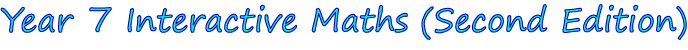1. Whole Numbers
2. Powers and Roots
3. Multiples and Factors
4. Fractions
5. Algebra
6. Decimals
7. Equations
8. Angles
9. Polygons
10. Percentages
11. Ratios
12. Length and Perimeter
13. Area of Plane Figures
14. Volume
15. Linear Relationships
16. Revision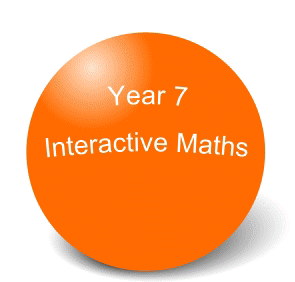The Year 7 Interactive Maths software is compatible with both Windows® and Mac® computers.  Discover more about Year 7 Interactive Maths by:

• Viewing the 15 chapter screen shots shown below that show step-by-step solutions in G S Rehill's proven writing style.  Students use the solutions after answering questions from the interactive exercises to identify mistakes in their working and to reinforce concepts, reasoning or mathematical laws.
• Clicking Mathematics Software Tutorials to watch 2 tutorials that show how students use the mathematics software.From Chapter 1: Whole Numbers, Exercise 16: Order of Operations.From Chapter 2: Powers and Roots, Exercise 13: Descending Order.From Chapter 3: Multiples and Factors, Exercise 5: Factors.From Chapter 4: Fractions, Exercise 4: Fractions Involving Addition.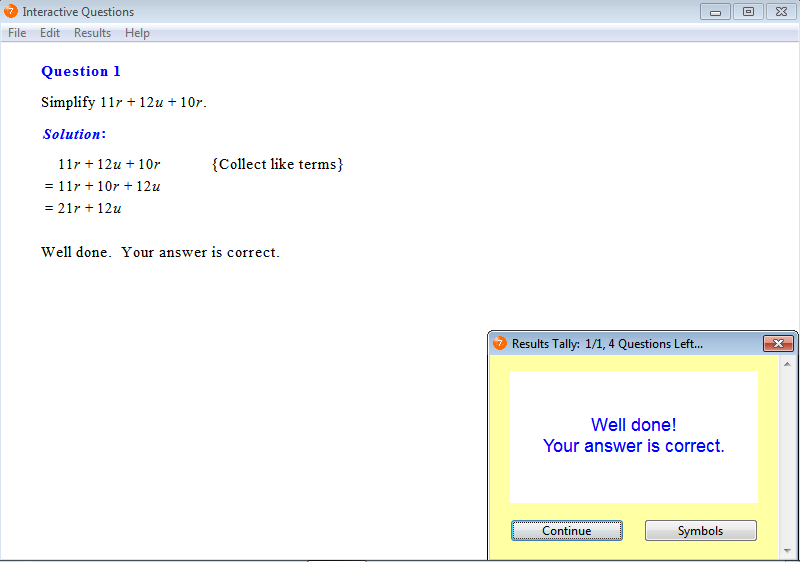From Chapter 5: Algebra, Exercise 12: Addition and Subtraction of Like Terms.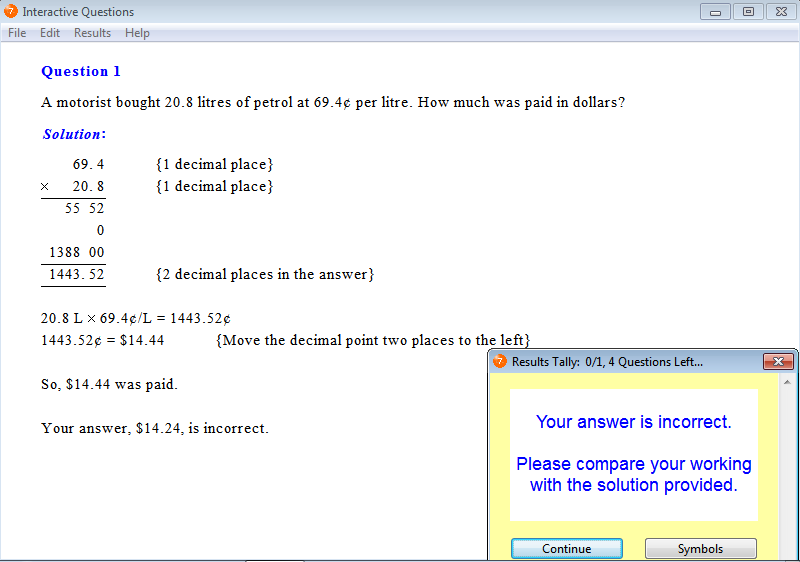From Chapter 6: Decimals, Exercise 15: Problem Solving.From Chapter 7: Equations, Exercise 13: Solving Word Problems.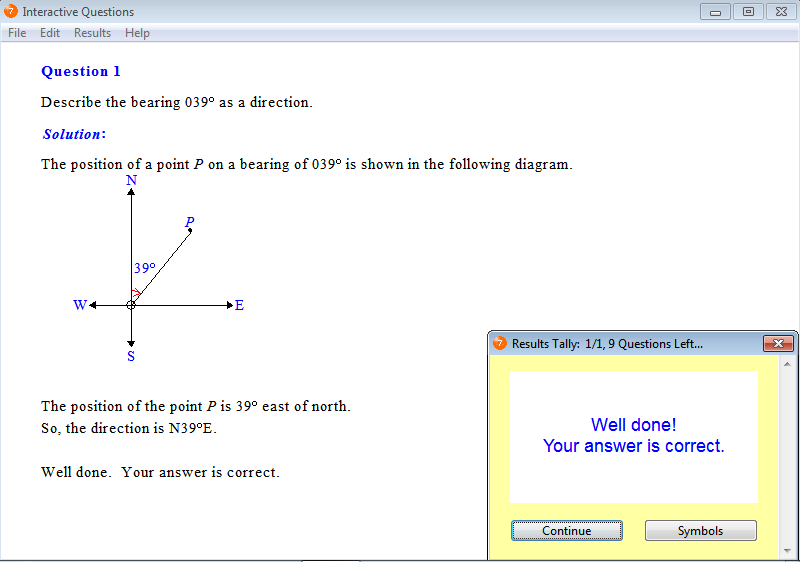From Chapter 8: Angles, Exercise 11: Direction.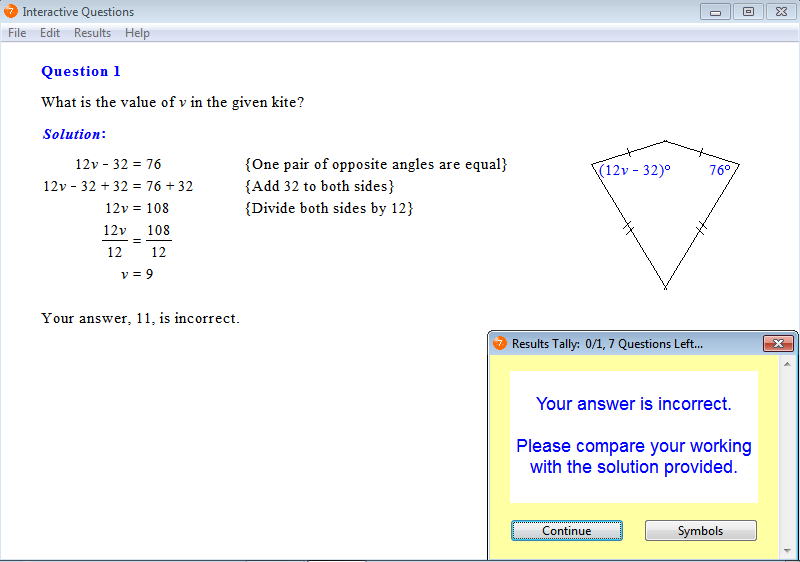From Chapter 9: Polygons, Exercise 12: Kites and Rhombuses.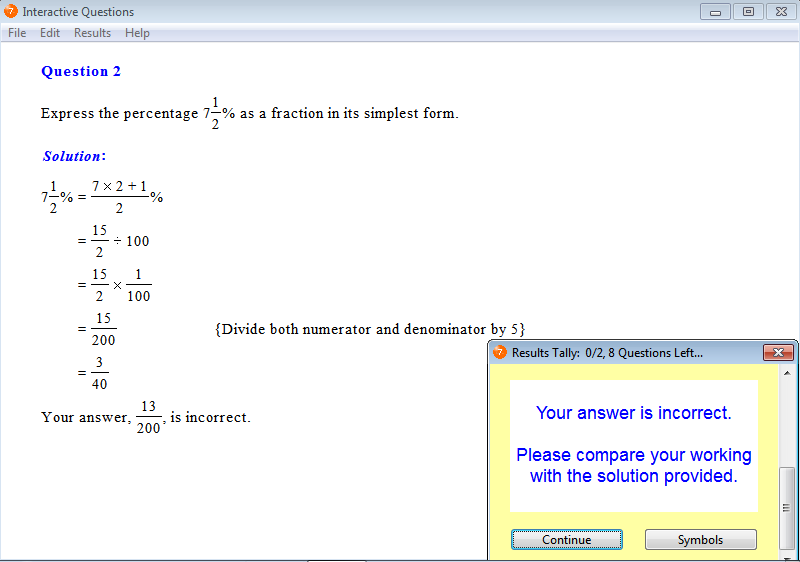From Chapter 10: Percentages, Exercise 2: Changing a Percentage to a Fraction.From Chapter 11: Ratios, Exercise 1: Comparing Quantities.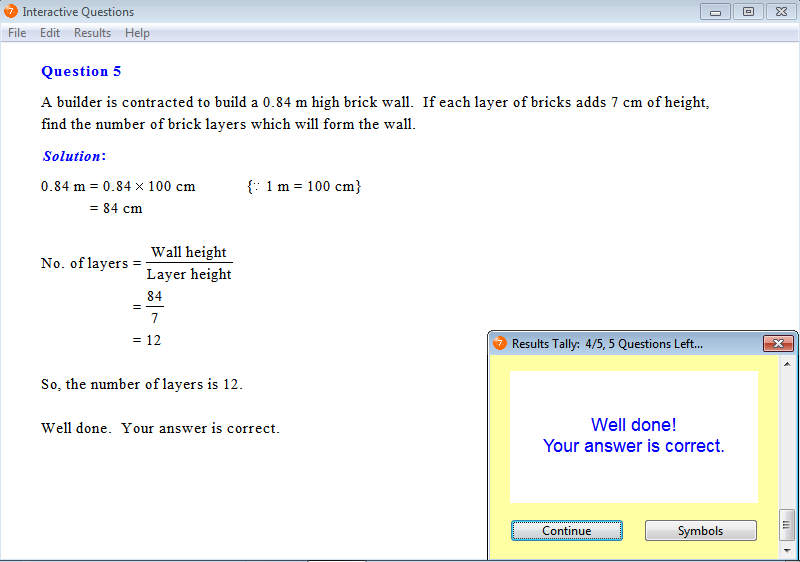From Chapter 12: Length and Perimeter, Exercise 10: Working with Metric Units of Length.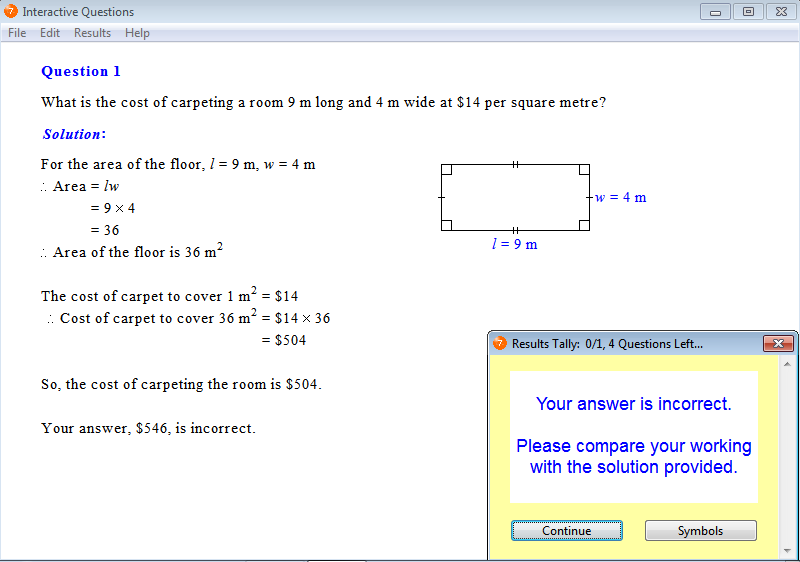From Chapter 13: Area of Plane Figures, Exercise 7: Problem Solving.From Chapter 14: Volume, Exercise 1: Finding Volume by Counting the Number of Cubes.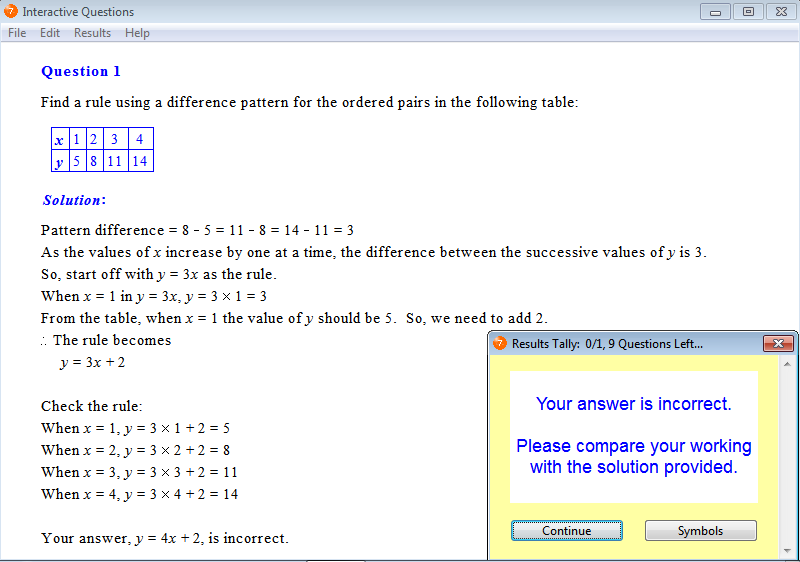From Chapter 15: Linear Relationships, Exercise 7: Modelling Linear Relationships with a Difference Pattern.

Parents can order a 12-month Homework Licence for \$19.95 (AUD) per student by clicking this order button: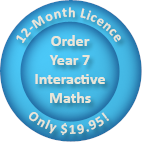Tutors may order Tutor Licences online that feature the interactive exercises as well as worksheets, tests and solution sheets.